# 3 Strategy

We begin with a free'' gauge theory, described by a Lagrangian action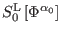, invariant under some gauge transformations(60)

and consider the problem of constructing consistent interactions among the fields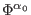such that the couplings preserve both the field spectrum and the original number of gauge symmetries. This matter is addressed by means of reformulating the problem of constructing consistent interactions as a deformation problem of the solution to the master equation corresponding to the free'' theory [39,40]. Such a reformulation is possible due to the fact that the solution to the master equation contains all the information on the gauge structure of the theory. If a consistent interacting gauge theory can be constructed, then the solution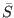to the master equation associated with the free'' theory,, can be deformed into a solution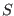,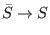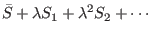(61)(62)

of the master equation for the deformed theory(63)

such that both the ghost and antifield spectra of the initial theory are preserved. The symboldenotes the antibracket. Equation ( 56) splits, according to the various orders in the coupling constant (or deformation parameter)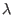, into the equivalent tower of equations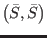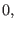(64)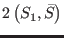(65)(66)(67)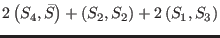0 (68)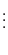(69)

Equation (57) is fulfilled by hypothesis. The next one requires that the first-order deformation of the solution to the master equation,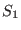, is a cocycle of the free'' BRST differential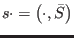. However, only cohomologically nontrivial solutions to (58) should be taken into account, as the BRST-exact ones can be eliminated by (in general nonlinear) field redefinitions. This means thatpertains to the ghost number zero cohomological space of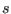,, which is generically nonempty due to its isomorphism to the space of physical observables of the free'' theory. It has been shown in [39,40] (on behalf of the triviality of the antibracket map in the cohomology of the BRST differential) that there are no obstructions in finding solutions to the remaining equations, namely, ( 59), (60) and so on. However, the resulting interactions may be nonlocal, and there might even appear obstructions if one insists on their locality. The analysis of these obstructions can be done with the help of cohomological techniques. As it will be seen below, all the interactions in the case of the model under study turn out to be local.

Ashkbiz Danehkar
2018-03-26